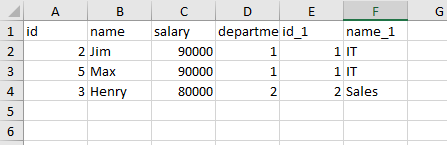# How to get max salary from each department

Hello Community,

How to get max salary from each department id from datatable?
Input_Data.xlsx (11.4 KB)

Any suggestions would be really appreciated.

HI,

Hope the following sample helps you.

``````dtResult = dt.AsEnumerable.GroupBy(Function(r) Int32.Parse(r("departmentid").ToString)).Select(Function(g) dtResult.LoadDataRow({g.key,g.Max(Function(r) Int32.Parse(r("salary").ToString))},False)).CopyToDataTable()
``````

Sample20220905-8.zip (13.0 KB)

Regards,

It missed the second salary which is same as max salary. It should have also printed.

HI,

Do you mean as the following?``````dt = dt.AsEnumerable.GroupBy(Function(r) Int32.Parse(r("departmentid").ToString)).SelectMany(Function(g) g.Where(Function(r) Int32.Parse(r("salary").ToString)= g.Max(Function(r2) Int32.Parse(r2("salary").ToString)))).CopyToDataTable()
``````

Sample20220905-8v2.zip (11.0 KB)

Regards,

Hi,

The following will work.

``````dtResult = dt.AsEnumerable.GroupBy(Function(r) Int32.Parse(r("departmentid").ToString)).SelectMany(Function(g) g.Where(Function(r) Int32.Parse(r("salary").ToString)= g.Max(Function(r2) Int32.Parse(r2("salary").ToString)))).Select(Function(r) dtResult.LoadDataRow({r("name_1"),r("name"),r("salary")},False)).CopyToDataTable()
``````

Sample20220905-8v3.zip (11.5 KB)

Regards,